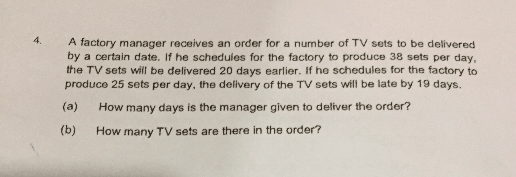# QuestionAppreciate help on this question. Thanks

This is a Group and Remainder question.

1. If 1 day produce 38 sets,  total = 38u. But extra = 20 × 38 = 760.

2. If 1 day produce 25 sets, total = 25u. But short of 19 × 25 = 475.

3. The totals are to be equal.

 Group Remainder Total R1 38u – 760 38u – 760 R2 25u + 475 25u + 475

4.  If 38 sets per day, there will be 760 set extra on delivery date. So we minus off from the total. (Unless the factory close down for 20 days)

5. If 25 sets per day, we need another 19 days to produce 475 set. So we add to the 25u.

38u – 760 = 25u + 475

38u – 25u = 760 + 475

13u = 1235

a)

1u = 1235 ÷ 13 = 95 days.

b)

95 × 38 = 3610

2 Replies 0 Likes ✔Accepted Answer

Shd answer for part B be

(95 – 20) x 38

Bec producing 38 tv per day will give 20 extra days?

0 Replies 0 Likes

Thank you for the clear explaination

0 Replies 0 Likes